Things started off badly this week when I pulled my FabISP out of my backpack and found it like this: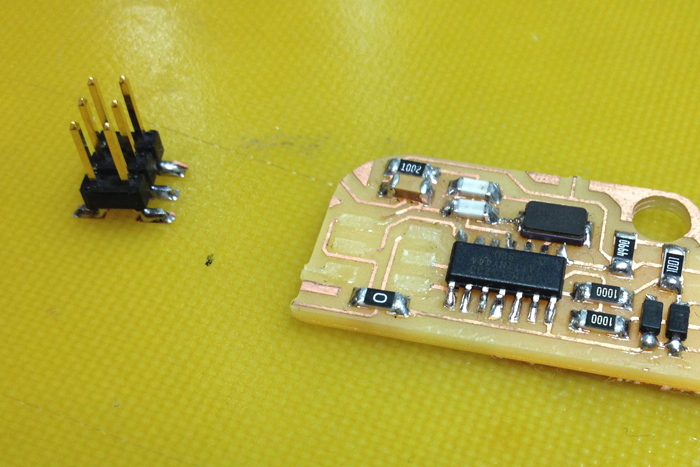It seemed like a waste to make a new board, so I threw a few jumper wires and some hot glue on it to get it up and running again.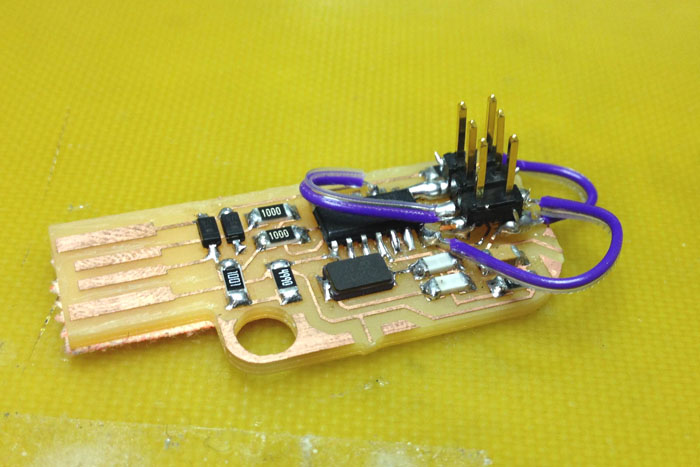I decided to drill through my hello world board and install through hole headers to make sure the connector stayed on for good. Our drill press has a vice mounted in it that you can move in x and y accurately, this was really helpful for getting exactly 0.1" spacing for the header.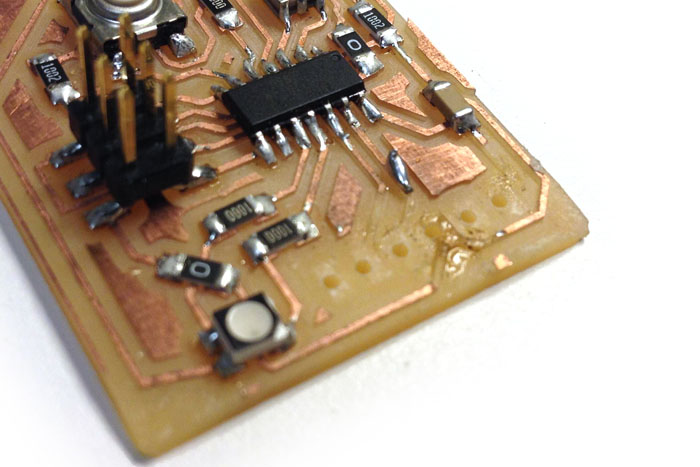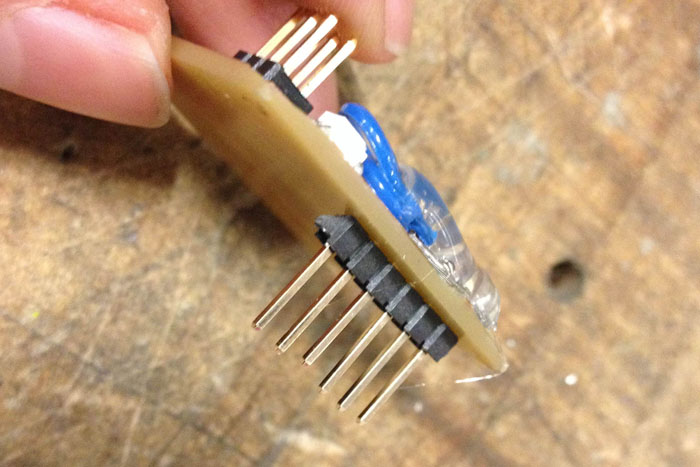Since the FTDI cables were in short supply this week, I also added a switch to my FabISP to optionally use it as a power supply for my hello world board. This made it so that I could program and run my board using only the FabISP connection. This made my workflow much nicer.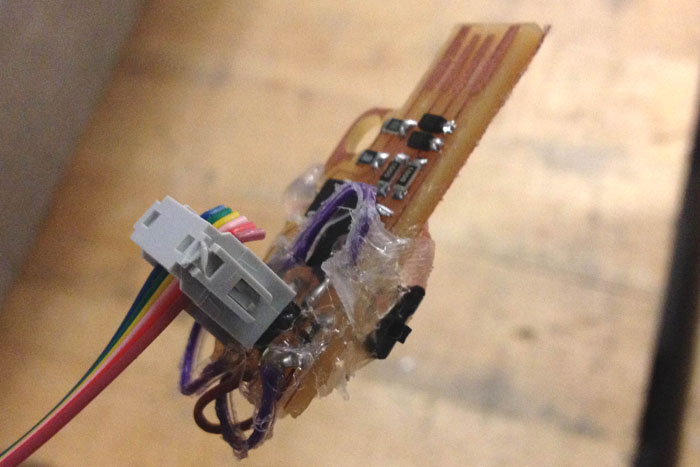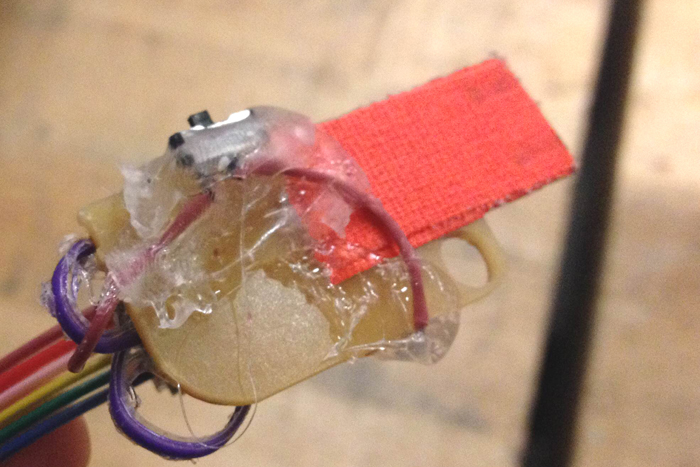Last week I noticed that the delay() function in the Arduino code was running extremely slowly on my ATtiny. I thought this was a timer issue, but now realize it was because the fuses weren't set properly to run the ATtiny at 20mHz. I followed the steps outlined here to set those using the Arduino IDE.

I re-uploaded the basic blink sketch from the examples section of Arduino and the timing is fixed!

Then I followed this tutorial to run Neil's echo.c code on my ATtiny (with one small edit).

First I downloaded hello.ftdi.44.echo.c and hello.ftdi.44.echo.c.make and navigated to my Downloads folder (or whatever folder you saved the files to) in the Terminal:

When I ran:

sudo make -f hello.ftdi.44.echo.c.make program-usbtiny

I got the following error: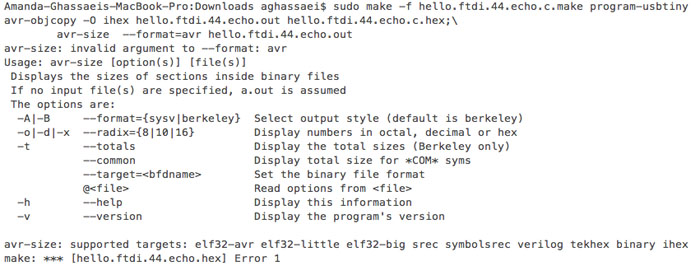I tried googling for the solution, but couldn't find much. I'd already installed Crosspack (and avrdude) and successfully programmed the FabISP so I know that avr-gcc was installed correctly on my computer. Finally, as I was about to email the class, I tried deleting the problem line entirely to see what would happen. hello.ftdi.44.echo.c.make now reads:

```PROJECT=hello.ftdi.44.echo
SOURCES=\$(PROJECT).c
MMCU=attiny44
F_CPU = 20000000

CFLAGS=-mmcu=\$(MMCU) -Wall -Os -DF_CPU=\$(F_CPU)

\$(PROJECT).hex: \$(PROJECT).out
avr-objcopy -O ihex \$(PROJECT).out \$(PROJECT).c.hex;\

\$(PROJECT).out: \$(SOURCES)
avr-gcc \$(CFLAGS) -I./ -o \$(PROJECT).out \$(SOURCES)

program-bsd: \$(PROJECT).hex
avrdude -p t44 -c bsd -U flash:w:\$(PROJECT).c.hex

program-dasa: \$(PROJECT).hex
avrdude -p t44 -P /dev/ttyUSB0 -c dasa -U flash:w:\$(PROJECT).c.hex

program-avrisp2: \$(PROJECT).hex
avrdude -p t44 -P usb -c avrisp2 -U flash:w:\$(PROJECT).c.hex

program-avrisp2-fuses: \$(PROJECT).hex
avrdude -p t44 -P usb -c avrisp2 -U lfuse:w:0x5E:m

program-usbtiny: \$(PROJECT).hex
avrdude -p t44 -P usb -c usbtiny -U flash:w:\$(PROJECT).c.hex

program-usbtiny-fuses: \$(PROJECT).hex
avrdude -p t44 -P usb -c usbtiny -U lfuse:w:0x5E:m

program-dragon: \$(PROJECT).hex
avrdude -p t44 -P usb -c dragon_isp -U flash:w:\$(PROJECT).c.hex
```

(I deleted the line avr-size --mcu=\$(MMCU) --format=avr \$(PROJECT).out )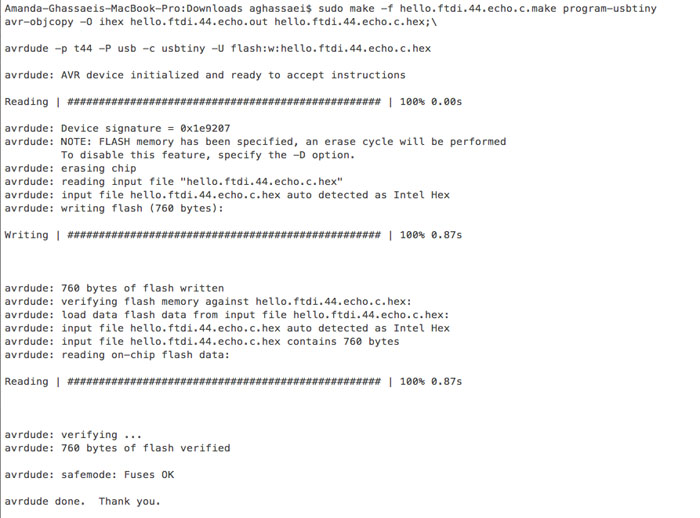To my astonishment, this actually fixed the problem and I was able to successfully connect to my board using the Arduino IDE's Serial Monitor.I read through the ATtiny44's datasheet to understand the layout of the ports/pins on the chip and figure out how to do timer interrupts. It turns out timer interrupts on the ATtiny are almost identical to the 328P (the chip on an Arduino) except that the ATtiny only has two timers. I borrowed code from an old Instructables post of mine about structuring timer interrupts on Arduino.

Section 9 of the ATtiny introduces all the types of interrupts that are possible on the chip.I'm using Timer/Counter Compare Match (CTC) interrupts in my code (sections 11 and 12 of the datasheet go into more detail about implementation). In this type of interrupt, the ATiny increments an internal counter (TCNT0 for timer 0 and TCNT1 for timer 1) at a frequency given by:

clock speed of ATtiny / prescaler

The prescalers for each timer can be set by setting bits on TCCR0B (for timer 1) and TCCR1B (for timer 2).

prescaler table for timer 0: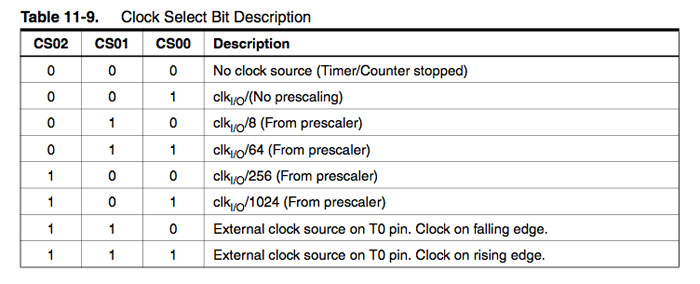prescaler table for timer 1: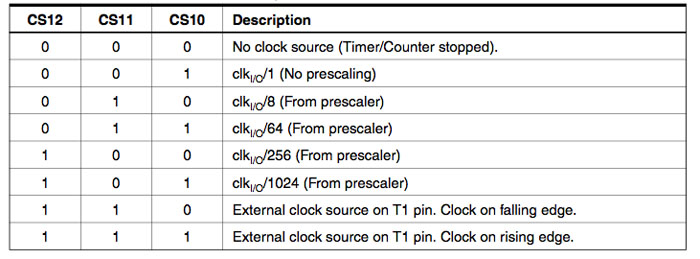so setting

TCCR0B |= (1 << CS01);

will give me a prescaler of 8 for timer 0.

TCCR1B |= (1 << CS10) |(1 << CS11);

will give me a prescaler of 64 for timer 1.

When the ATtiny's internal counter increments above a value stored by OCR0A (for timer0) or OCR1A (for timer 1), the interrupt routine will execute. At this point the internal counter is reset to zero. You can calculate the value of OCR0A for a desired interrupt frequency with the following equation:

(clock speed) / (desired interrupt frequency*prescaler) - 1

An important thing to note is that timer 0 is an 8 bit timer, so OCR0A and TCNT0 cannot store values greater than 256. You should use a higher prescaler instead. Timer 1 is a 16 bit timer, so OCR1A and TCNT1 can go as high as 65536.

I used timer interrupts to do software PWM on my RGB led since I wasn't able to hook up all my pins to a hardware PWM. I programmed by board with the following code in Arduino:

```//helloWorld

byte buttonPin = 7;
byte redLED = 2;
byte blueLED = 3;
byte greenLED = 2;

//green led has pwm - use it
byte currentGreenIntensity = 0;
boolean greenIntensityDirection = true;

byte appState = 0;

//button debouncing variables
boolean currentButtonState = false;
boolean lastButtonState = false;
boolean debouncedButtonState = false;
byte buttonDebounceMax = 10;
byte buttonDebounceTimer = buttonDebounceMax;

//softPWM variables
volatile byte redLEDIntensity = 0;
volatile byte greenLEDIntensity = 0;
volatile byte blueLEDIntensity = 0;
volatile byte softPWMCounter = 0;

volatile byte currentColor = 1;

void setup(){

//set inputs/outputs
DDRA = 0;
DDRA = (1<<redLED)|(1<<blueLED);
DDRB = 0;
DDRB = (1<<greenLED);

//turn LEDs off
turnOffAllLEDS();

cli();//disable interrupts

TCCR0A = 0;// set entire TCCR0A register to 0
TCCR0B = 0;// same for TCCR0B
TCNT0  = 0;//initialize counter value to 0
// set compare match register for 200khz increments
OCR0A = 99;// = (20*10^6) / (200000*1) - 1 (must be <256 for 8 bit timer)
// turn on CTC mode
TCCR0A |= (1 << WGM01);
// Set CS00 bit for 1 prescaler
TCCR0B |= (1 << CS00);
// enable timer compare interrupt
TIMSK0 |= (1 << OCIE0A);

TCCR1A = 0;// set entire TCCR1A register to 0
TCCR1B = 0;// same for TCCR1B
TCNT1  = 0;//initialize counter value to 0
// set compare match register for 1hz increments
OCR1A = 194;// = (20*10^6) / (100*1024) - 1 (must be <65536 for 16 bit timer)
// turn on CTC mode
TCCR1B |= (1 << WGM12);
// Set CS10 and CS12 bits for 1024 prescaler
TCCR1B |= (1 << CS12) | (1 << CS10);
// enable timer compare interrupt
TIMSK1 |= (1 << OCIE1A);

sei();//enable interrupts
}

void loop(){

appState = debounceButton(appState);

switch(appState){
case 0://red
redLEDIntensity = 255;
greenLEDIntensity = 0;
blueLEDIntensity = 0;
break;
case 1://green
redLEDIntensity = 0;
greenLEDIntensity = 255;
blueLEDIntensity = 0;
break;
case 2://blue
redLEDIntensity = 0;
greenLEDIntensity = 0;
blueLEDIntensity = 255;
break;
break;
case 4://pause at current color
break;
case 5:
turnOffAllLEDS();
break;
}
}

byte debounceButton(byte _appState){

currentButtonState = (PINA>>buttonPin)&1;

//button debouncing
if (currentButtonState != lastButtonState && currentButtonState != debouncedButtonState){//if current button state is different than last button state and current debounced state
if (currentButtonState){//if the button is currently pressed
debouncedButtonState = currentButtonState;
_appState = getNextAppState(appState);
} else {
buttonDebounceTimer = buttonDebounceMax;//reset debounce counter
}
} else if (currentButtonState == lastButtonState && currentButtonState != debouncedButtonState){//if current state same as last but different that debounced
buttonDebounceTimer--;
if (buttonDebounceTimer == 0){
debouncedButtonState = currentButtonState;
if (currentButtonState){//if the button is currently pressed
_appState = getNextAppState(appState);
}
}
}

lastButtonState = currentButtonState;

return _appState;
}

byte getNextAppState(byte state){
state++;
if (state > 5) return 0;
return state;
}

void turnOffAllLEDS(){
//these are common anode leds, so we have to remove their connection to ground to turn them off

redLEDIntensity = 0;
greenLEDIntensity = 0;
blueLEDIntensity = 0;

PORTA |= (1 << redLED);
PORTA |= (1 << blueLED);
PORTB |= (1 << greenLED);
}

//softPWM
ISR(TIM0_COMPA_vect ){//timer0 interrupt 200kHz
softPWMCounter++;//let this overflow to zero

if (redLEDIntensity>softPWMCounter){
PORTA &= ~(1 << redLED);
} else {
PORTA |= (1 << redLED);
}
if (greenLEDIntensity>softPWMCounter){
PORTB &= ~(1 << greenLED);
} else {
PORTB |= (1 << greenLED);
}
if(blueLEDIntensity>softPWMCounter){
PORTA &= ~(1 << blueLED);
} else {
PORTA |= (1 << blueLED);
}

}

//set new intensity vals
ISR(TIM1_COMPA_vect ){//timer1 interrupt 100Hz
switch(currentColor){
case 1://red
{
if (redLEDIntensity == 0){
currentColor = 2;
break;
}
redLEDIntensity--;
greenLEDIntensity++;
break;
}
case 2://green
{
if (greenLEDIntensity == 0){
currentColor = 3;
break;
}
greenLEDIntensity--;
blueLEDIntensity++;
break;
}
case 3://blue
{
if (blueLEDIntensity == 0){
currentColor = 1;
break;
}
blueLEDIntensity--;
redLEDIntensity++;
break;
}
}
}
}```

Then I refactored my program in c and uploaded it via the terminal. Here is the code:

```//softPWM

#include <avr/io.h>
#include <avr/pgmspace.h>
#include <avr/interrupt.h>

typedef int bool;
#define true 1
#define false 0

#define buttonPin 7
#define redLED 2
#define blueLED 3
#define greenLED 2

//green led has pwm - use it
int currentGreenIntensity = 0;
bool greenIntensityDirection = true;

int appState = 0;

//button debouncing variables
bool currentButtonState = false;
bool lastButtonState = false;
bool debouncedButtonState = false;
#define buttonDebounceMax 10;
int buttonDebounceTimer = buttonDebounceMax;

//softPWM variables
volatile int redLEDIntensity = 0;
volatile int greenLEDIntensity = 0;
volatile int blueLEDIntensity = 0;
volatile int softPWMCounter = 0;

volatile int currentColor = 1;

int getNextAppState(int state){
state++;
if (state > 5) return 0;
return state;
}

int debounceButton(int _appState){

currentButtonState = (PINA>>buttonPin)&1;

//button debouncing
if (currentButtonState != lastButtonState && currentButtonState != debouncedButtonState){//if current button state is different than last button state and current debounced state
if (currentButtonState == true){//if the button is currently pressed
debouncedButtonState = currentButtonState;
_appState = getNextAppState(appState);
} else {
buttonDebounceTimer = buttonDebounceMax;//reset debounce counter
}
} else if (currentButtonState == lastButtonState && currentButtonState != debouncedButtonState){//if current state same as last but different that debounced
buttonDebounceTimer--;
if (buttonDebounceTimer == 0){
debouncedButtonState = currentButtonState;
if (currentButtonState == true){//if the button is currently pressed
_appState = getNextAppState(appState);
}
}
}

lastButtonState = currentButtonState;

return _appState;
}

void turnOffAllLEDS(){
//these are common anode leds, so we have to remove their connection to ground to turn them off

redLEDIntensity = 0;
greenLEDIntensity = 0;
blueLEDIntensity = 0;

PORTA |= (1 << redLED);
PORTA |= (1 << blueLED);
PORTB |= (1 << greenLED);
}

//softPWM
ISR(TIM0_COMPA_vect){//timer0 interrupt 200kHz
softPWMCounter++;//let this overflow to zero
if (softPWMCounter>255){
softPWMCounter = 0;
}

if (redLEDIntensity>softPWMCounter){
PORTA &= ~(1 << redLED);
} else {
PORTA |= (1 << redLED);
}
if (greenLEDIntensity>softPWMCounter){
PORTB &= ~(1 << greenLED);
} else {
PORTB |= (1 << greenLED);
}
if(blueLEDIntensity>softPWMCounter){
PORTA &= ~(1 << blueLED);
} else {
PORTA |= (1 << blueLED);
}

}

//set new intensity vals
ISR(TIM1_COMPA_vect){//timer1 interrupt 100Hz
switch(currentColor){
case 1://red
{
if (redLEDIntensity == 0){
currentColor = 2;
break;
}
redLEDIntensity--;
greenLEDIntensity++;
break;
}
case 2://green
{
if (greenLEDIntensity == 0){
currentColor = 3;
break;
}
greenLEDIntensity--;
blueLEDIntensity++;
break;
}
case 3://blue
{
if (blueLEDIntensity == 0){
currentColor = 1;
break;
}
blueLEDIntensity--;
redLEDIntensity++;
break;
}
}
}
}

int main(void) {

//set inputs/outputs
DDRA = 0;
DDRA = (1<<redLED)|(1<<blueLED);
DDRB = 0;
DDRB = (1<<greenLED);

//turn LEDs off
turnOffAllLEDS();

cli();//disable interrupts

TCCR0A = 0;// set entire TCCR0A register to 0
TCCR0B = 0;// same for TCCR0B
TCNT0  = 0;//initialize counter value to 0
// set compare match register for 200khz increments
OCR0A = 99;// = (20*10^6) / (200000*1) - 1 (must be <256 for 8 bit timer)
// turn on CTC mode
TCCR0A |= (1 << WGM01);
// Set CS00 bit for 1 prescaler
TCCR0B |= (1 << CS00);
// enable timer compare interrupt
TIMSK0 |= (1 << OCIE0A);

TCCR1A = 0;// set entire TCCR1A register to 0
TCCR1B = 0;// same for TCCR1B
TCNT1  = 0;//initialize counter value to 0
// set compare match register for 1hz increments
OCR1A = 194;// = (20*10^6) / (100*1024) - 1 (must be <65536 for 16 bit timer)
// turn on CTC mode
TCCR1B |= (1 << WGM12);
// Set CS10 and CS12 bits for 1024 prescaler
TCCR1B |= (1 << CS12) | (1 << CS10);
// enable timer compare interrupt
TIMSK1 |= (1 << OCIE1A);

sei();//enable interrupts

while (1) {
appState = debounceButton(appState);
switch(appState){
case 0://red
redLEDIntensity = 255;
greenLEDIntensity = 0;
blueLEDIntensity = 0;
break;
case 1://green
redLEDIntensity = 0;
greenLEDIntensity = 255;
blueLEDIntensity = 0;
break;
case 2://blue
redLEDIntensity = 0;
greenLEDIntensity = 0;
blueLEDIntensity = 255;
break;
break;
case 4://pause at current color
break;
case 5:
turnOffAllLEDS();
break;
}

}
}```

Here is the make file:

```PROJECT=softPWM
SOURCES=\$(PROJECT).c
MMCU=attiny44
F_CPU = 20000000

CFLAGS=-mmcu=\$(MMCU) -Wall -Os -DF_CPU=\$(F_CPU)

\$(PROJECT).hex: \$(PROJECT).out
avr-objcopy -O ihex \$(PROJECT).out \$(PROJECT).c.hex

\$(PROJECT).out: \$(SOURCES)
avr-gcc \$(CFLAGS) -I./ -o \$(PROJECT).out \$(SOURCES)

program-bsd: \$(PROJECT).hex
avrdude -p t44 -c bsd -U flash:w:\$(PROJECT).c.hex

program-dasa: \$(PROJECT).hex
avrdude -p t44 -P /dev/ttyUSB0 -c dasa -U flash:w:\$(PROJECT).c.hex

program-avrisp2: \$(PROJECT).hex
avrdude -p t44 -P usb -c avrisp2 -U flash:w:\$(PROJECT).c.hex

program-avrisp2-fuses: \$(PROJECT).hex
avrdude -p t44 -P usb -c avrisp2 -U lfuse:w:0x5E:m

program-usbtiny: \$(PROJECT).hex
avrdude -p t44 -P usb -c usbtiny -U flash:w:\$(PROJECT).c.hex

program-usbtiny-fuses: \$(PROJECT).hex
avrdude -p t44 -P usb -c usbtiny -U lfuse:w:0x5E:m

program-dragon: \$(PROJECT).hex
avrdude -p t44 -P usb -c dragon_isp -U flash:w:\$(PROJECT).c.hex
```

I named the files softPWM.make and softPWM.c and ran the following in the terminal:

sudo make -f softPWM.make program-usbtiny